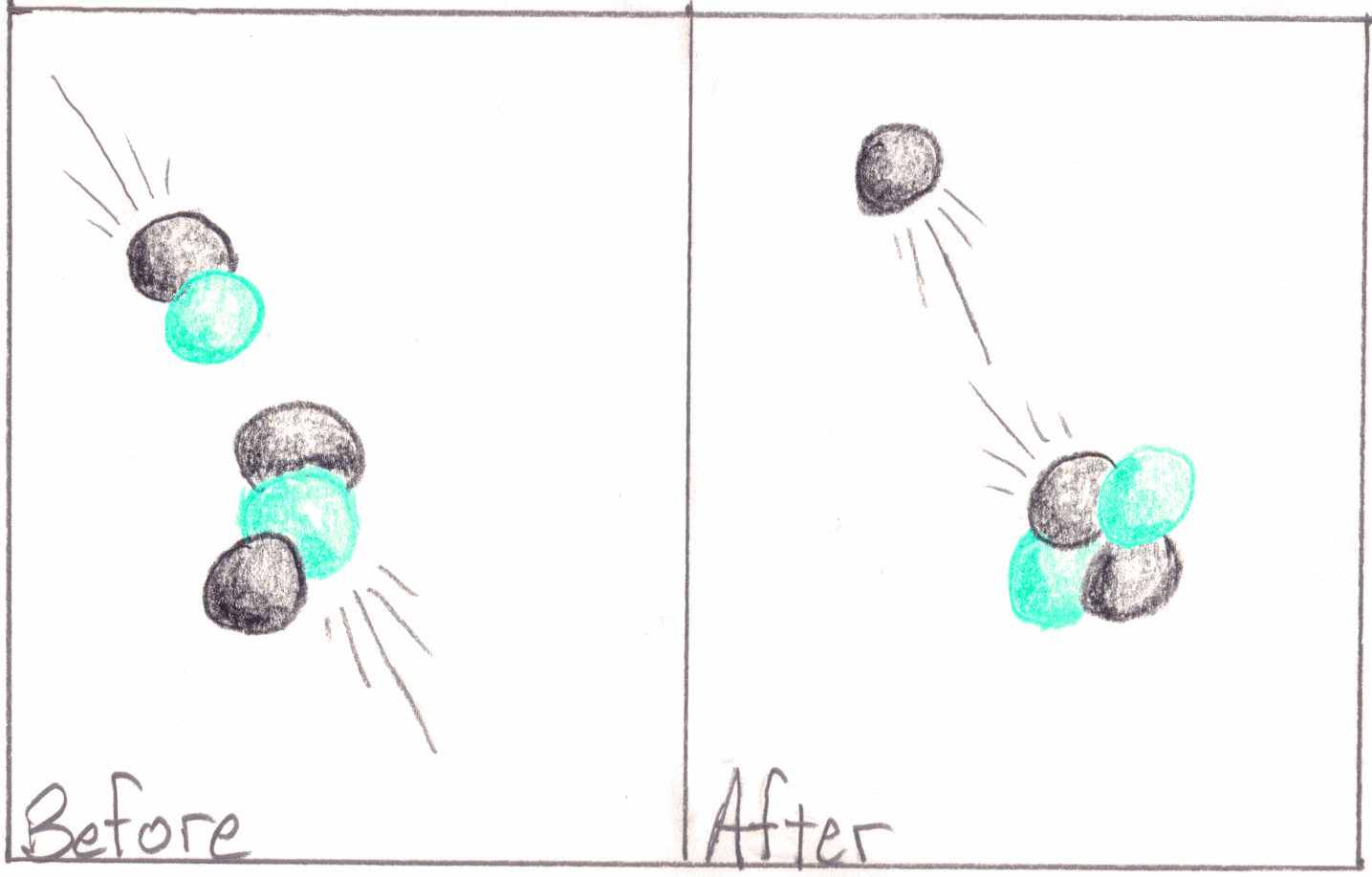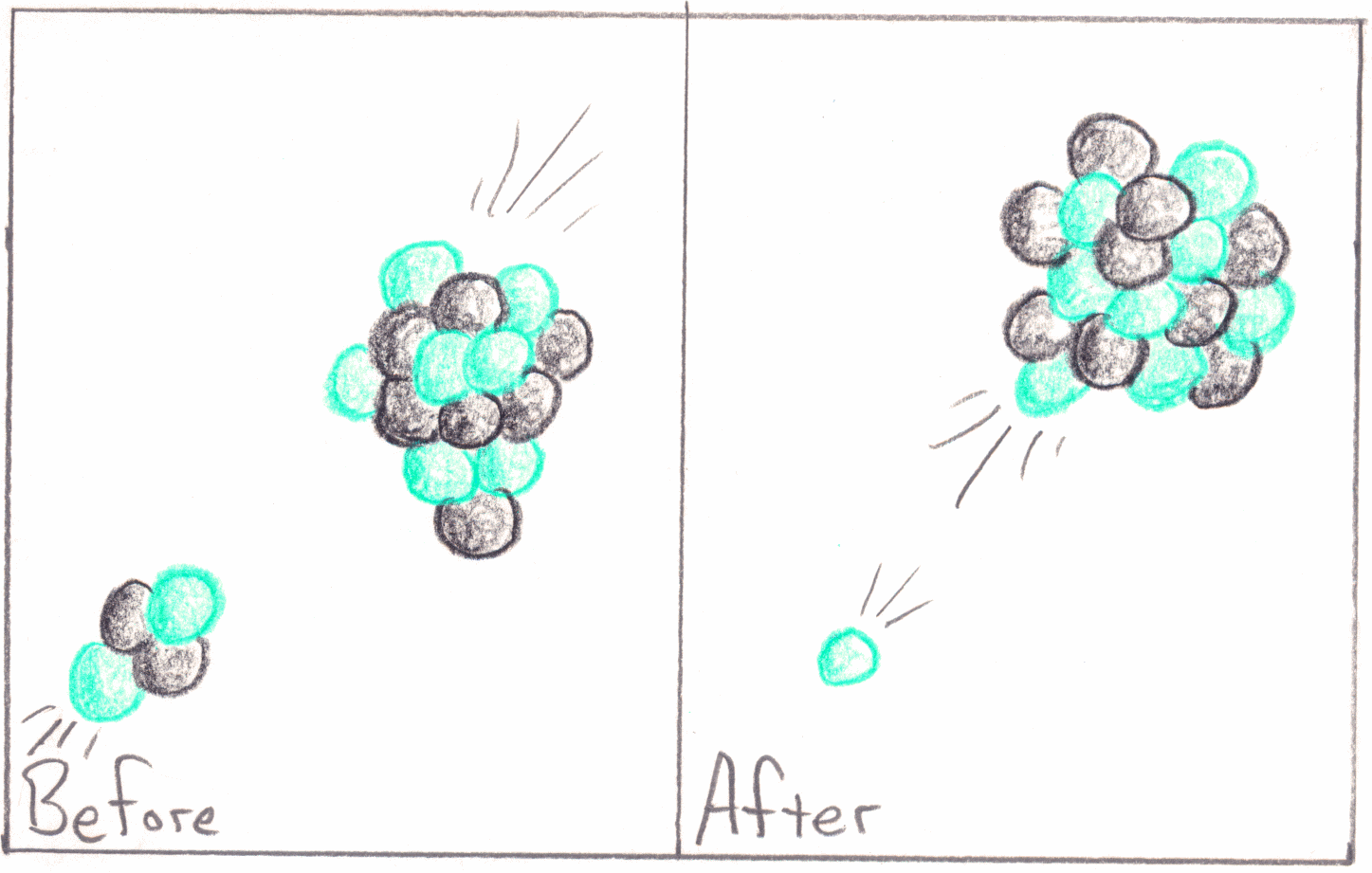Date:
Class:

## POGIL Activity: Nuclear Equations

I am in the process of completely re-writing this lesson. The original sole introduction is here: Intro to Nuclear Chem. It can be used as a summary and review document for the lessons in the new sequence.
This activity is the third activity in the sequence. Here are links to the others:
Alpha and Beta Decay with Simulations

This work should be preceded by two things. First, students should have an opportunity to explore electric forces on a macroscopic scale by using pieces of plastic that obtain an electric charge when rubbed. Second, students should have a basic understanding of light and the electromagnetic spectrum.
A full table of the isotopes: Wikipedia.
Here are some links with visuals and charts for various concepts that need to be covered in lecture/discussion:
Nuclear Notation and Forces
Fundamental Forces
Neutron Excess Table of Isotopes Diagram (this chart is useful for pointing out where on the chart isotopes that are beta, positron/EC, or alpha emitters)

Also, show this table of radioactive decay modes with animations
And the Interactive Chart of Nuclides, NuDat 2.4, from the National Nuclear Data Center, Brookhaven National Laboratory.
Here is a great glossary of nuclear terminology.
PhET Simulation about Balloons and Static Electricity
“Travoltage” PhET Simulation
PhET Alpha Decay Simulation
PhET Beta Decay Simulation PhET Simulation for Nuclear Fission
See the accompanying assignments: Alpha and Beta Radiation
and Positron Emission and Electron Capture
Lab: The Geiger Counter

### Nuclear Symbols

When learning about atomic structure students usually also learn about isotope notation. To give a full description of the make-up of an isotope it isn’t necessary to write out a sentence. A simple symbol will do. The symbol works like this: AZX, where A stands for the mass number and gives the sum of the number of protons and neutrons in the nucleus. And Z stands for the atomic number, which equals the number of protons in a nucleus. It does not give the number of electrons, which can vary. The letter X stands in for any atomic symbol in the periodic table.Fill in the following table with the required information about the isotopes in the picture at left.
 Name Symbol p+ n0 Mass hydrogen-1 1 1H 1 0 1 hydrogen-2 2 1H
Model 1
1. Which color is used for protons in Model 1? Which color is used for neutrons?
2. What is the meaning of the letter ‘Z’ when speaking about atomic nuclei?
3. What is the definition of the atomic mass number (A)?
1. Write a mathematical expression that relates mass number (A) to atomic number (Z) and the number of neutrons (n0).
2. What is the meaning of the number that often follows the name of an element?
3. When the name of an element is followed by a number (as in calcium-40), what does it refer to, an isotope or an element? Why?

Review
1. What is alpha decay?
2. What is the atomic symbol for an alpha-particle?
3. What is beta decay?
4. What is the atomic symbol for a beta-particle?
1. What is positron decay?
2. What is the atomic symbol for a positron?
3. What is electron-capture decay?
4. What is the atomic symbol for an electron?

page break

### Nuclear Decay Equations

Nuclear equations are used communicate about natural radioactive decay and other nuclear events such as fission, fusion, and transmutation. All nuclear equations follow a simple pair of rules. First, the sum of all atomic numbers on one side of the equation must equal the sum of all atomic numbers on the other side of the equation. Second, the sum of all mass numbers on one side of the equation must equal the sum of all mass numbers on the other side of the equation. Model 2 shows some example nuclear decay equations.

1. 20884Po  —> 4  2He  + 20482Pb
2. 14 6C  —> 14  7N  + 0-1β
3. 5025Mn  —> 5024Cr  + 0+1β+
4. 8136Kr  + 0-1e   —> 8135Br
Model 2
1. What does the arrow mean in these equations?
2. Describe what is happening in nuclear equation number one, at left.
3. What is happening in equation number 2?

1. What is happening in equation number 3?
2. What is happening in equation number 4?
3. Write a nuclear equation for the alpha decay of americium-243.
4. Write a nuclear equation for the beta decay of oxygen-19.
5. Write a nuclear equation for the positron decay of bromine-68.
6. Write a nuclear equation for the electron capture decay of nickel-56.
1. Given the decay mode and a daughter nucleus, how would you figure out the identity of the parent nucleus?
2. Write a balanced nuclear equation for an alpha decay that produces radon-218 as the daughter.
3. Write a balanced nuclear equation for a beta decay that produces xenon-131 as the daughter.
4. Write a balanced nuclear equation for a positron decay that produces chromium-50 as the daughter.
5. Write a balanced nuclear equation for an electron capture decay that produces uranium-233 as the daughter.

page break

### Other Nuclear Equations

Nuclear equations can also be used to describe other interesting things besides nuclear decay. For example, all life on Earth owes its existence to atomic fusion reactions happening deep in the core of the Sun. Fusion is when atomic nuclei smash into one another and combine along with the release of an enormous amount of energy. Nuclear electric power stations and nuclear bombs both operate by taking advantage of the process of atomic fission. Atomic fission is when an atom breaks apart into two large nuclear pieces. Finally, transmutation is when atomic nuclei are smashed together to form new nuclei. Lead cannot be made into gold this way but it is possible to change small numbers of atoms of one element into another. Model 3 shows several illustrations of these processes along with the nuclear equations which describe them.Nuclear Fusion Artificial Transmutation 21H + 31H → 42He + 10n0 42He + 147N → 178O + 11HDescribe what happened in the fusion illustration above and at left. What is the sum of mass numbers on the left side of the arrow in the fusion equation? Does it equal the sum on the other side? What is the sum of atomic numbers on the left side of the arrow in the fusion equation? Does it equal the sum on the other side? Nuclear Fission 23592U + 10n0 → 14156Ba + 9236Kr + 310n0
Model 3

1. Describe what happened in the fission illustration above.
2. What is the meaning of the coefficient in front of the symbol for a neutron?
3. Check the sums of atomic and mass numbers in the fission equation. Do they work out as equal on both sides?
1. What makes a fusion reaction different from a fission reaction?
2. Describe what happened in the transmutation illustration above.
3. Check the sums of atomic and mass numbers in the transmutation equation. Do they work out as equal on both sides?

page break

Complete the following exercises to practice writing and completing nuclear decay, fusion, fission, and transmutation equations.

For the following exercises, fill in the missing particle and give a name to what is happening. Identify whether it is a type of nuclear decay, fission, fusion, or transmutation. Only one particle is missing from each equation. Space has been provided both before and after the arrow but you must determine where the missing particles belong.

1. 23490Th                → 42He
2. 20179Au                → 0–1e
3. 4727Co                → 4726Fe
(there are two possible correct answers; give both)
4.                 → 7333As + 0+1e+
5.                 → 42He + 22286Rn
1.                 2713Al → 3015P + 10n0
2.                 126C + 24696Cm → 410n0
3.                 21H + 21H → 10n0
4.                 10n09037Rb + 14355Cs + 310n0
5.                 23994Pu + 10n014858Ce + 210n0

Each of the following exercises describes a nuclear change. Write a correct and balanced nuclear equation for each one.

1. A neptunium-237 nucleus undergoes alpha-decay.
2. Write an equation for the positron decay of gallium-59.
3. An iron-58 nucleus is hit by a bismuth-209 nucleus to form meitnerium-266 and a neutron.
1. Write an equation for the electron capture decay of polonium-200.
2. A plutonium-239 nucleus absorbs a neutron and fissions to release three neutrons, a krypton-81 nucleus and one other atomic nucleus.
3. Magnesium-24 is the result of the beta-decay of another isotope of another element. Identify the element and write the nuclear equation.
1. On a separate piece of paper create an outline that contains all of the main points that you believe you were meant to learn by doing this activity. Do it with bullets or with headings and sub-headings (A., B., 1., 2., etc.) Work with your group on this part and consult with your teacher before moving on to the next part of the activity.
2. On a separate piece of paper use your outline to write a short paragraph that summarizes all of the main points you wrote above. Do this part completely independently but share your work with your group members after you are finished. Make any changes or additions you find necessary to make a final draft of this information. Type up this draft to turn in as the recorder’s report.
Here is a summary of the information students are meant to learn by doing this activity:
Summary of POGIL Nuclear Equations

Last updated: Jan 24, 2023        Home